## ‘Math Suks’ ~ Jimmy Buffett

Need to relax after studying? Close to wanting to “burn your textbook”? This super chill and somewhat cheesy song may help! Coming to you from Jimmy Buffett, the singer has a relaxed, island/ska like vibe to his music.  The lyrics come close to sounding like an after school special until the words “Math Sucks” are sung over and over again.  Enjoy! 🙂

‘Math Suks’ by Jimmy Buffet Lyrics:

If necessity is the mother of invention
Then I’d like to kill the guy who invented this
The numbers come together in some kind of a third dimension
A regular algebraic bliss.

Any two plus two will never get you five.
There are fractions in my subtraction and x don’t equal y
But my homework is bound to multiply.

Math suks math suks
I’d like to burn this textbook, I hate this stuff so much.
Math suks math suks
Sometimes I think that I don’t know that much
But math suks.

I got so bored with my homework, I turned on the TV.
The beauty contest winners were all smiling through their teeth.
Then they asked the new Miss America
Hey babe can you add up all those bucks?
She looked puzzled, then just said
“Math Suks”.

Math suks math suks
You don’t even have to spell it,
All you have to do is yell it…
Math suks math suks
Sometime times I think that I don’t know that much
But math suks.

Geometry, trigonometry and if that don’t tax your brain
There are numbers to big to be named
Numerical precision is a science with a mission
And I think it’s gonna drive me insane.

Parents fighting with their children, and the Congress can’t agree
Teachers and their students are all jousting constantly.
Management and labor keep rattling old sabers
Quacking like those Peabody ducks.

Math suks math suks
You don’t even have to spell it,
All you have to do is yell it…
Math suks math suks
Sometime times I think that I don’t know that much
But math suks

## Simultaneous Equations: Algebra

In need of a bit of review on Simultaneous Equations?  Well, now is your chance! Learn how to solve these confusing bad mama jamma’s in three different ways and choose which one works best for you!

I’m also excited to introduce my new page for MathSux!  Hope these new set of videos help.  Let me know if you have any more questions in the comments.  Happy calculating! 🙂

Need more MathSux in your life?  Follow us on these great sites!

## Geometry: Congruent Triangles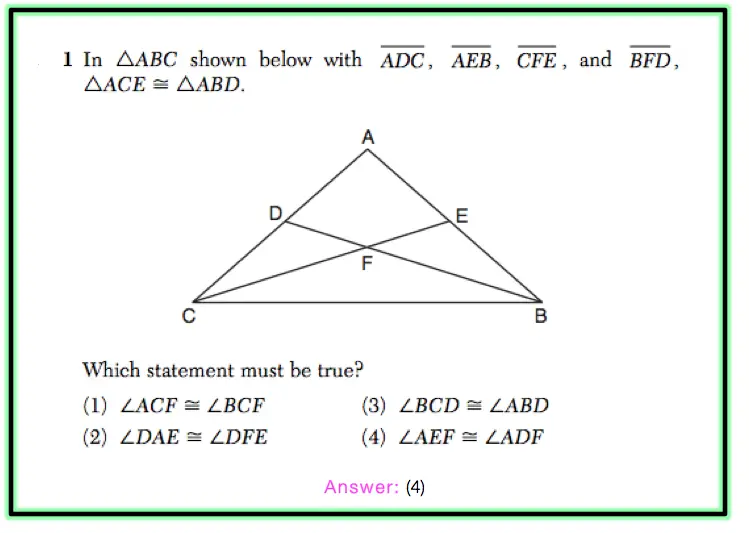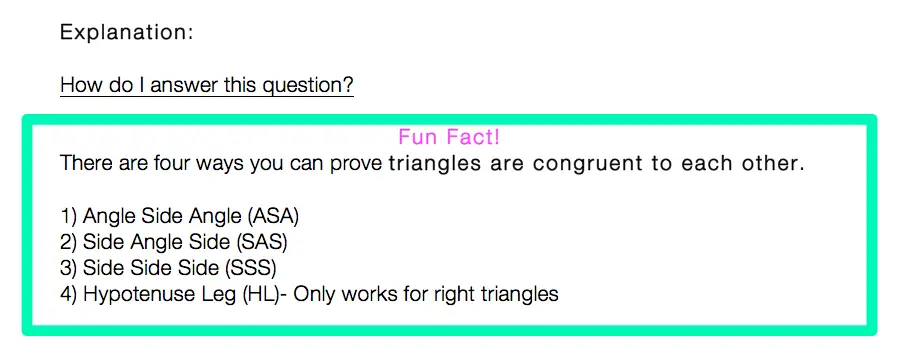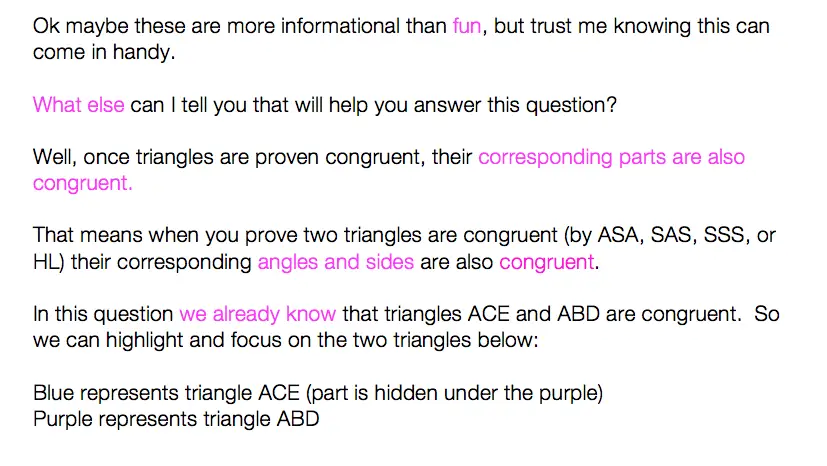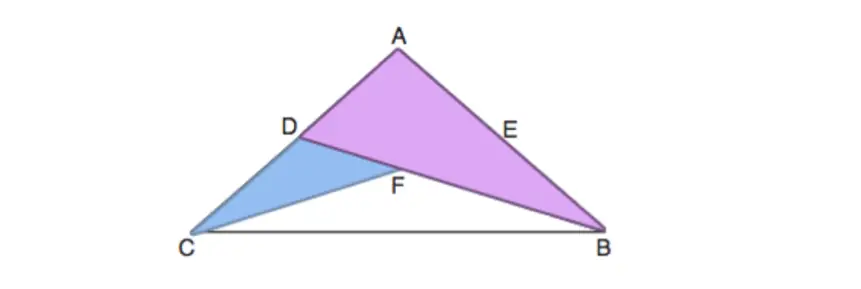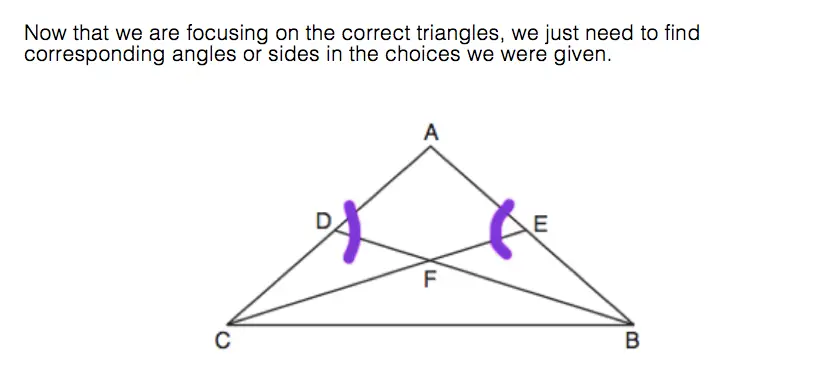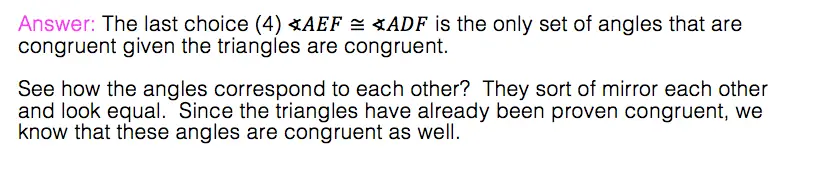Not bad, eh? If there is anything you have questions on please don’t hesitate to ask! 🙂 If you are looking to test out your skills on this topic even more, check out more challenging questions here.

And for more of me and my nerdy math jokes take a look at MathSux on

## Solving Trigonometric Equations: Algebra 2/Trig.

Howdy math friends! In this post, we are going to learn about solving trigonometric equations algebraically.  This will combine our knowledge of algebra and trigonometry into one beautiful question! For more on trigonometric functions and right triangle trigonometry check out this post here.

Solving trigonometric equations. Sound complicated? Well, you are correct, that does sound complicated. Is it complicated? Hopefully, you won’t fund it that way after you’ve seen this example. We are going to do this step by step in the following regents question:

## How do I answer this question?

The questions want us to solve for x.

Step 1: Pretend this was any other equation and you wanted to solve for x. Thinking that way, we can move radical 2 to the other side.

Step 3: Now we need a value for x. This is where I turn to my handy dandy reference triangle. (This is a complete reference tool that you should memorize know). For more on special triangles, check out this post here.

Step 5: Notice that cosine is positive in Quadrants I and IV. That means there are two values that x can be (one in each quadrant). We already have x=45º from Quadrant I. In order to get that other value in Quadrant IV, we must subtract 360º-45º=315º giving us our other value.

Does this make sense? Great! 🙂 Is it clear as mud? I have failed. But I have not given up (and neither should you). Ask more questions, look for the spots where you got lost, do more research and never give up! 🙂

Hopefully you enjoyed my short motivational speech. For more encouraging words and math, check out MathSux on the following websites! Sign up for FREE math videos, lessons, practice questions, and more. Thanks for stopping by and happy calculating! 🙂

## PieceWise Functions NYS Regents: Algebra

Greetings math friends, students, and teachers I come in peace to review this piecewise functions NYS Regents question.  Are they pieces of functions? Yes. Are they wise?  Ah, yeah sure, why not? Let’s check out this piecewise functions NYS Regents question below and happy calculating! 🙂

1 value satisfies the equation because there is only one point on the graph where f(x) and g(x) meet.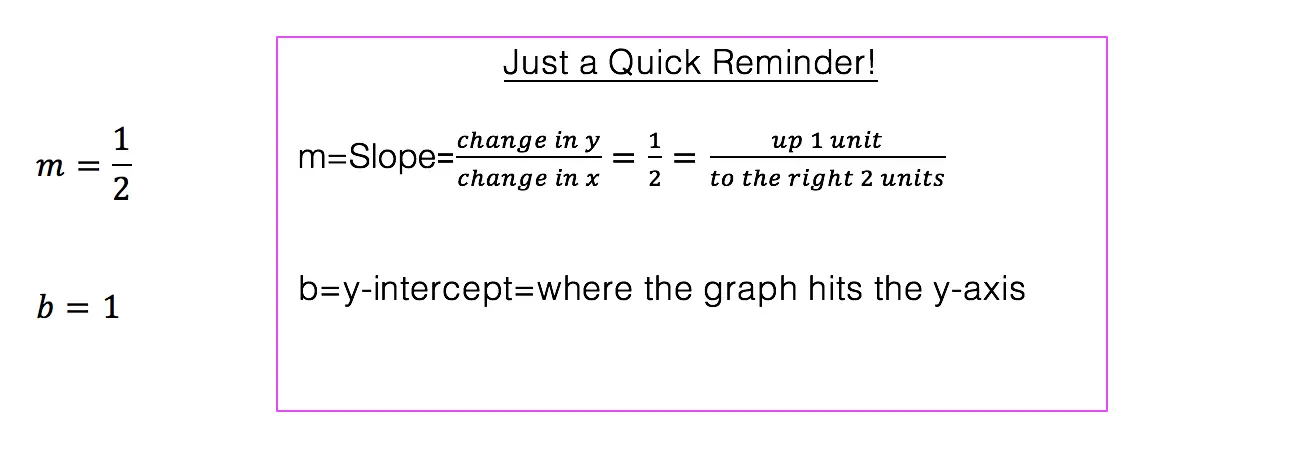*Note: For an in-depth review on how to graph an equation of a line step by step, check out this post here.

Does the above madness make sense to you? Great!

Need more of an explanation? Keep going! There is a way to understand the above mess.

Looking at this piece-wise function: We want to graph the function 2x+1 but only when the x-values are less than or equal to negative 1.

We also want to graph 2-x^2 but only when x is greater than the negative one.

One way to organize graphing each piece of a piecewise function is by making a chart.

1. Lets start by making a chart for the first part of our function 2x+1:

Is it all coming back to you now?  Need more practice on piece-wise functions?Check out this link here and happy calculating! 🙂

Also, if you’re looking to nourish your mind or you know, procrastinate a bit check out and follow MathSux on these websites!

## Top 5 Favorite Math Websites

As we all love technology (I’m assuming since it has brought you here), I figured I should share my top favorite math sites.  These are great resources for learning things you don’t understand, practicing for an upcoming test or if you’re like me, it’s great for re-learning/teaching purposes.  Yes, even I forget how to do things and these websites are a great help.  I know the anticipation is getting to you so I’ll just get to the good stuff now. Here are my top 5 favorite math websites:

1. Khan Academy : This is a non profit over in Silicon Valley.  They have a great range of subjects (k-college level)  and explain everything via easy to follow videos.
2. Regent’s Prep: The title is pretty self explanatory, but this helps with reviewing for the New York State Regent’s.  This site has a lot of great examples and answers.
3.  MathSux:  So fabulous, no explanation is required. 😀
4.  Knewton:  Pretty cool things are happening at this web address.  You can make an account, answer some questions and the questions will adjust according to your answers figuring out what you need help in.
5. Kahoot: Fast and easy quizzes that are good for an entire classroom or for studying alone.  Either way there are lots of bright and happy colors that make you forget you’re studying anything in the first place.

So those are the goods, the contraband, the info you can’t find anywhere else (all lies).  Hope you find these websites useful. Let me know if you end up using any of them.  🙂

## Factor by Grouping: Algebra 2/Trig.

Hey math friends! In this post, we are going to go over Factor by Grouping, one of the many methods for factoring a quadratic equation.  There are so many methods to factor quadratic equations, but this is a great choice, for when a is greater than 1. Also, if you need to review different types of factoring methods, just check out this link here.  Stay curious and happy calculating! 🙂 Before we go any further, let’s just take a quick look at what a quadratic equation is: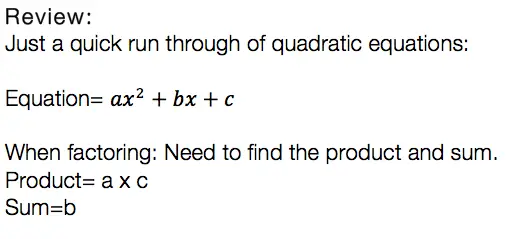Usually, we can just find the products, the sum, re-write the equation, solve for x, and be on our merry way.  But if you notice, there is something special about the question below. The coefficient “a” is greater than 1/. This is where factor by grouping comes in handy!Now that we know why and when we need to factor by grouping lets take a look at our Example: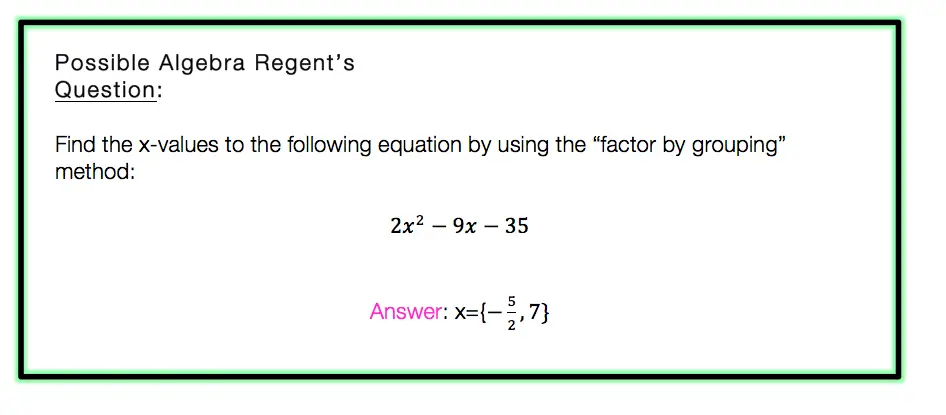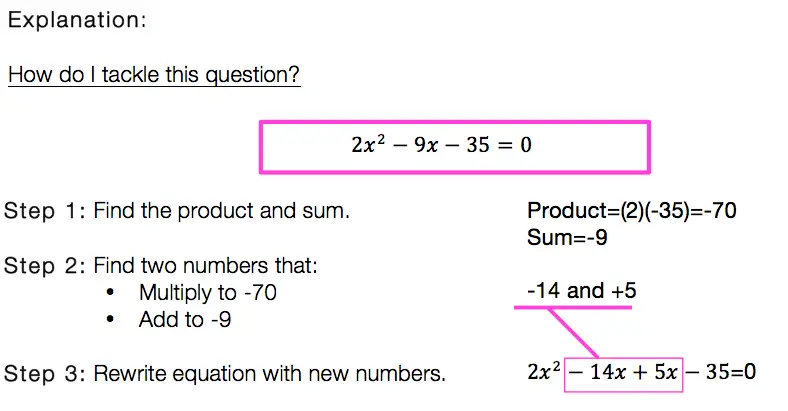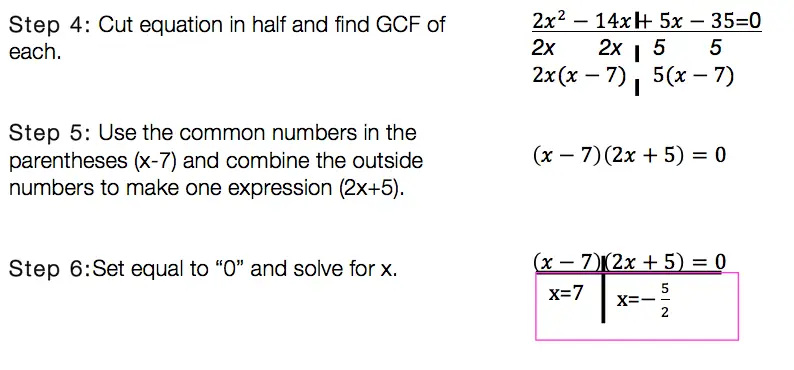Factor By Grouping: Hard to solve? No.  Hard to remember? It can be, just remember to practice, practice practice! Also, if you are in need of a review of other methods of factoring quadratic equations, click this link here.

Want more Mathsux?  Don’t forget to check out our Youtube channel and more below! And if you have any questions, please don’t hesitate to comment. Happy Calculating! 🙂

## Recursive Formula: Algebra

Howdy math peeps! In this post, we are going to go over the recursive formula step by step by reviewing a Regent’s question. Yes, it is the recursive formula jam, well at least it’s my kind of jam.  These things may look weird, confusing, and like a “what am I doing?” moment, but trust me they are no so bad!

How do I answer this question?

At first glance, all of these answer choices may look exactly the same. The first thing I would want to do with this question is to identify how all of theses answer choices are different. Take a look below:

What is the Recursive Formula?

A Recursive Formula is a formula that forms a sequence based on the previous term value. All this means is that it uses a formula to form a sequence-based pattern.

Let’s go through each choice to identify the answer:

Our goal is to test out each choice given, until we get the desired sequence:

Still have questions about recursive formulas? Check out more on recursive formulas here!

Still got questions? No problem! Check out the video above for more or try the NYS Regents question below, and please don’t hesitate to comment with any questions. Happy calculating! 🙂

## Solving Log Equations: Algebra 2/Trig.

Ahoy math friends! In this post, we are going to focus on solving log equations by solving this Regents questions step by step. We’ll answer this question right away! But if you need more of a review, keep reading and you will find what logarithms are, the different kinds of logarithms rules, and some simpler examples. Ready for our first example?! Check it out below:

## How do I Answer this Question?

Step 1: Let’s re-write the equation to get rid of the “log.”

Step 2: Solve for x in our new equation (5x-1)(1/3)=4

If the above answer makes sense to you, great! If not, that’s ok too, keep reading for a review on solving log equations.

## What are Logarithms?

Logarithms are inverses of exponential equations. Take a look below for a clearer picture.

Basic Formula:

Logarithms can be re-written to get rid of the word “log.” This makes them easier to solve and understand.

Log Rules:

There are a few rules you have to memorize get used to with practice. These rules are used when solving for x in different kinds of algebraic log problems:

Still got questions?  No problem! Check out the videos below and the post here for more on logarithms! Also don’t forget to subscribe below to get the latest FREE math videos, lessons, and practice questions from MathSux. Thanks for stopping by and happy calculating! 🙂